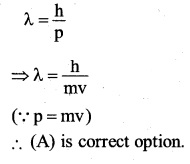# 2nd PUC Physics Question Bank Chapter 11 Dual Nature of Radiation and Matter

## Karnataka 2nd PUC Physics Question Bank Chapter 11 Dual Nature of Radiation and Matter

### 2nd PUC Physics Dual Nature of Radiation and Matter NCERT Text Book Questions and Answers

Question 1.
Find the
(a) Maximum frequency, and
(b) Minimum wavelength of X-rays produced by 30 kV electrons.
V=30kV = 30×103 V
(a) The maximum frequency of X-rays produced is given by,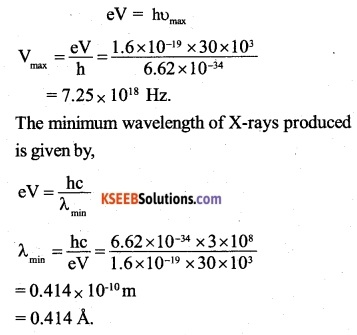Question 2.
The work function of caesium metal is 2.14 eV. When light of frequency 6 x 1014Hz is incident on the metal surface, photoemission of electrons occurs. What is the
(a) Maximum kinetic energy of the emitted electrons,
(b) Stopping potential, and
(c) Maximum speed of the emitted photoelectrons?
υ=6×1014Hz
ω =2.14eV = 2.14 x 1.6 x 10-19J
(a) The maximum kinetic energy of the emitted electrons is given by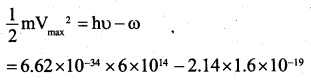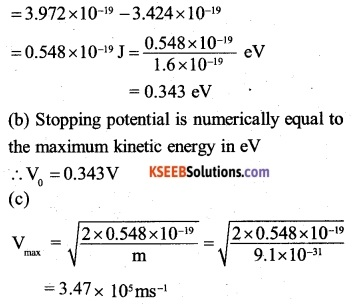Question 3.
The photoelectric cut-off voltage in a certain experiment is 1.5 V. What is the maximum kinetic energy of photo electrons emitted?
V0 = 1.5 V
∴ Maximum kinetic energy of the emitted electrons,
$$\frac { 1 }{ 2 } m{ V }_{ max }^{ 2 }=e{ V }_{ 0 }=1.5j=15eV$$Question 4.
Monochromatic light of wavelength 632.8 nm is produced by a helium-neon laser. The power emitted is 9.42 raW.

(a) Find the energy and momentum of each photon in the light beam,

(b) How many photons per second, on the average, arrive at a target irradiated by this beam? (Assume the beam to have uniform cross-section which is less than the target area), and

(c) How fast does a hydrogen atom have to travel in order to have the same momentum as that of the photon?
λ= 632.8 nm= 632 x 10-9m
power, P = 9.42 mW = 9.42 x 10-3 W
(a) Energy of each photon in the light beam,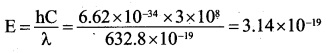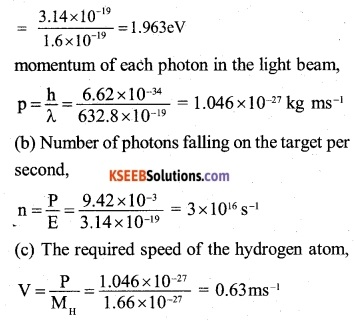Question 5.
The energy flux of sunlight reaching the surface of the earth is 1.388 x 103 W/m2. How many photons (nearly) per square metre are incident on the Earth per second? Assume that the photons in the sunlight have an average wavelength of 550 nm.
Energy flux of sunlight reaching the surface of the earth,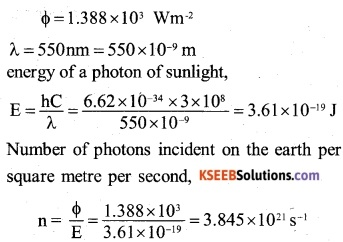Question 6.
In an experiment on photoelectric effect, the slope of the cut-off voltage versus frequency of incident light is found to be 4.12 x 10-15 V s. Calculate the value of Planck’s constant.
Slope of the cut off voltage versus frequency of incident light graph
= 4.12 x 10-15 Vs
but the slope of the graph = $$\frac { h }{ e }$$
h = e x slope of the graph
= 1.6 x 1019 x 4.12 x 10-15 = 6.592 x10-34JsQuestion 7.
A 100W sodium lamp radiates energy uniformly in all directions. The lamp is located at the centre of a large sphere that absorbs all the sodium light which is incident on it. The wavelength of the sodium light is 589 nm.
(a) What is the energy per photon associated with the sodium light?
(b) At what rate are the photons delivered to the sphere?
Power of the lamp, P = 100 W
λ =589nm=589×10-9m
(a) Therefore, energy of a photon of sodium lamp light,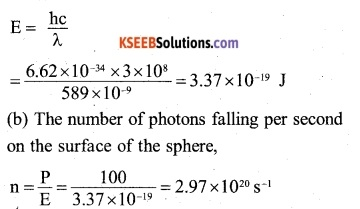Question 8.
The threshold frequency for a certain metal is 3.3 x 1014 Hz. If light of frequency
8.2 x 1014 Hz is incident on the metal, predict the cutoff voltage for the photoelectric emission.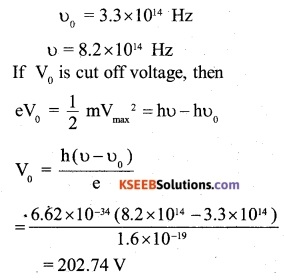Question 9.
The work function for a certain metal is 4.2 eV. Will this metal give photoelectric emission for incident radiation of wavelength 330 nm?
X, = 330 nm = 330 x 10-9m
∴ Energy of a photon of incident light,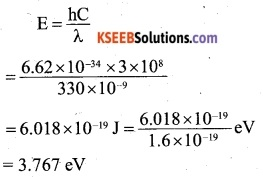Since the energy of the photon of incident light is less than the work function for the metal, photoelectric emission will not take place.Question 10.
Light of frequency 7.21 x 1014 Hz is incident on a metal surface. Electrons with a maximum speed of 6.0 x 105 m/s are ejected from the surface. What is the threshold frequency for photoemission of electrons?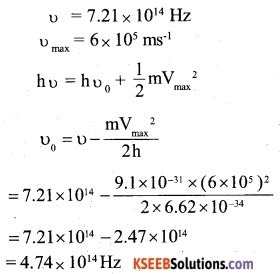Question 11.
Light of wavelength 488 nm is produced by an argon laser which is used in the photoelectric effect. When light from this spectral line is incident on the emitter, the stopping (cut-off) potential of photo electrons is 0.38 V. Find the work function of the material from which the emitter is made.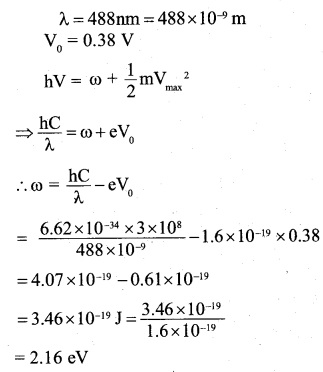Question 12.
Calculate the
(a) Momentum, and
(b) de Broglie wavelength of the electrons accelerated through a potential difference of 56 V.
V = 56 V
(a) When an electron is accelerated through a potential difference V, it acquires kinetic energy, which is given by, $$\frac { 1 }{ 2 }$$mV2 – eV Therefore, momentum of electron.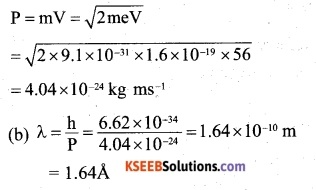Question 13.
What is the
(a) Momentum,
(b) Speed, and
(c) de Broglie wavelength of an electron .with kinetic energy of 120 eV.
V = 120eV
(a) When an electron is accelerated through a potential difference V, it acquires kinetic energy which is given by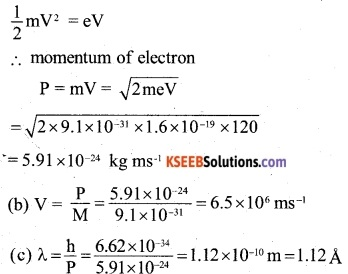Question 14.
The wavelength of light from the spectral emission line of sodium is 589 nm. Find the kinetic energy at which
(a) An electron, and
(b) A neutron, would have the same de Broglie wavelength.
λ = 589 nm= 589 x 109 m.
The de-Broglie wavelength of a particle having energy E is given by,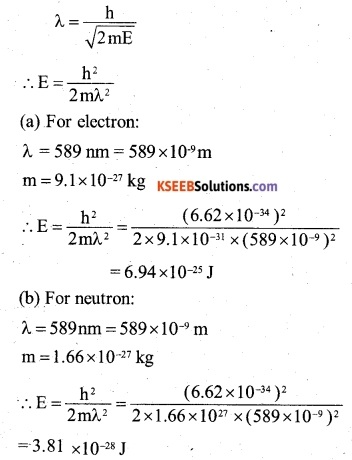Question 15.
What is the de Broglie wavelength of
(a) A  bullet of mass 0.040 kg travelling at the speed of 1.0 km/s,
(b) A ball of mass 0.060 kg moving at a speed of 1.0 m/s, and
(c) A dust particle of mass 1.0 x 10 9 kg drifting with a speed of 2.2 m/s?
The de-Broglie wavelength of a particle moving with a velocity V is given by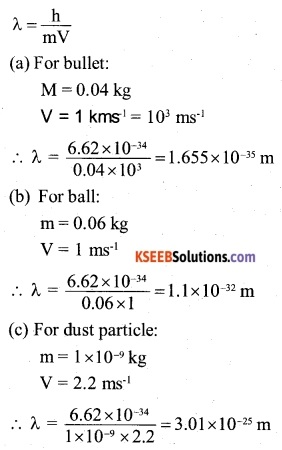Question 16.
An electron and a photon each have a wavelength of 1.00 nm. Find
(a) Their momenta,
(b) The energy of the photon, and
(c) The kinetic energy of electron.
h = 6.63 × 10-34 Js
λ= 1 nm= 10-9 m
(a) The electron and the photon will possess the same momentum, which is given by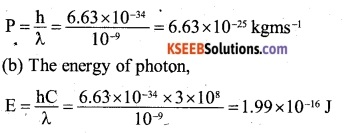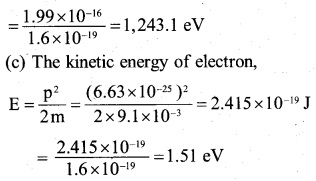Question 17.
(a) For what kinetic energy of a neutron will the associated de Broglie wavelength be 1.40 x 10-10m?
(b) Also find the de Broglie wavelength of a neutron, in thermal equilibrium with matter, having an average kinetic energy of (3/2) k T at 300 K.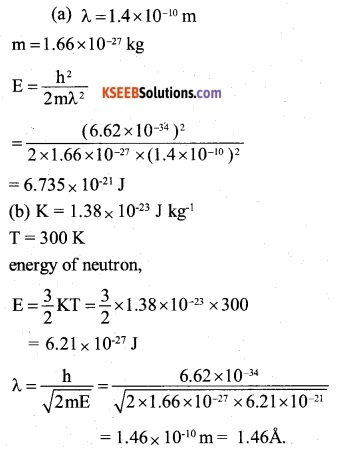Question 18.
Show that the wavelength of electromagnetic radiation is equal to the de Broglie wavelength of its quantum (photon).
Consider an electromagnetic radiation of wavelength λ and frequency υ. Then,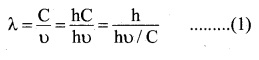But
$$\frac { h\upsilon }{ C } =P………………(2)\quad$$
P = momentum of the electromagnetic radiation. From equations (1) and (2), we have
$$\lambda =\frac { h }{ P }$$
Hence the wavelength of electromagnetic radiation is equal to the de-Broglie wavelength associated with its quantum.Question 19.
What is the de Broglie wavelength of a nitrogen molecule in air at 300 K? Assume that the molecule is moving with the root- mean square speed of molecules at this temperature. (Atomic mass of nitrogen = 14.0076 u)
K= 1.38 x 10-23Jkg-1
T = 300 K
Nitrogen is a diatomic gas
∴ Mass of the nitrogen gas molecule,
m= 2 x 14.0076 × 1027amu
= 2 x 14.0076 x 1027 = 4.65 ×10-26 kg.
The root mean square speed of the nitrogen gas molecule is given by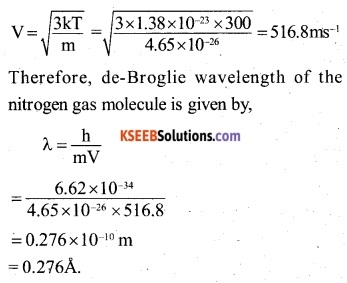### 2nd PUC Physics Dual Nature of Radiation and Matter Additional Exercises

Question 20.
(a) Estimate the speed with which electrons emitted from a heated emitter of an evacuated tube impinge on the collector maintained at a potential difference of 500 V with respect to the  emitter. Ignore the small initial speeds of, the electrons. The specific charge of the electron, i.e., its e/m is given to be 1.76 x 1011 C kg-1

(b) Use the same formula you employ in (a) to obtain electron speed for a collector potential of 10 MV. Do you see what is wrong? In what way is the formula to be modified?
(a) V = 500 V
e/m= 1.76×1011 C kg-1
Due to the applied potential difference between the emitter and the collector, an emitted electron gains kinetic energy, which is given by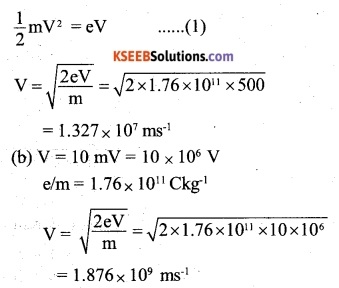Using the same formula as in (a), the speed of electrons at 10 mV comes out to be greater than the speed of light. Since no material particle can move with the speed of light, the result is clearly wrong.

In fact, equation (1) is valid for kinetic energy of the electron, so long as V/C < < 1. At 10 mV, the electrons attain a speed comparable to the speed of light i.e. become relativistic.

In the relativistic domain:- The mass of the electron having rest mass m() is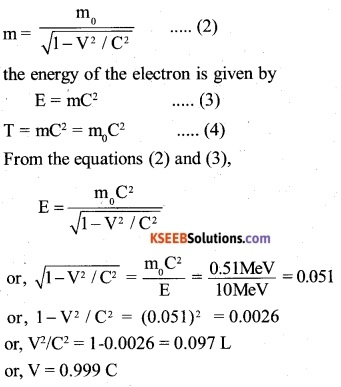Question 21.
(a) A monoenergetic electron beam with electron speed of 5.20 x 106 m s-1 is subject to a magnetic field of 1.30 x 10-4 T normal to the beam velocity. What is the radius of the circle traced by the beam, given e/m for electron equals 1.76 x 10nC kg-1.

(b) Is the formula you employ in (a) valid for calculating radius of the path of a 20 MeV electron beam? If not, in what way is it modified ?

[Note: Exercises 11.20(b) and 11.21(b) take you to relativistic mechanics which is beyond the scope of this book. They have been inserted here simply to emphasise the point that the formulas you use in part (a) of the exercises are not valid at very high speeds or energies. See answers at the end to know what ‘very high speed or energy’ means.]
(a) V = 5.2×106 ms1
B = 1.3 × 10-4
e/m=1.76 x 1011Ckg-1

When a magnetic field is applied normally to the path of electron, the force due to the field makes the electron to move along the circular path. The radius of the circular path is given by,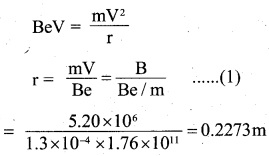The 10 MeV electron attain a speed comparable to the speed of light i.e. becomes relativistic. In the relativistic domain, the mass of an electron, having rest mass M0 is given by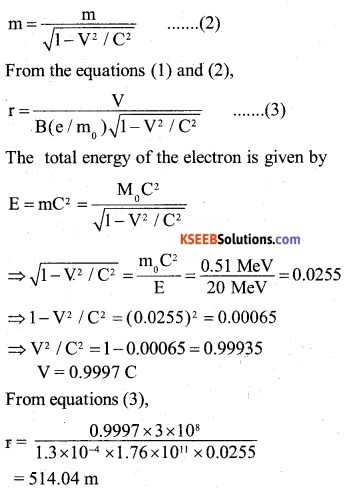Question 22.
An electron gun with its collector at a potential of 100 V fires out electrons in a spherical bulb containing hydrogen gas at low pressure (~10-2 mm of Hg). A magnetic field of 2.83 x 10-4 T curves the path of the electrons in a circular orbit of radius 12.0 cm. (The path can be viewed because the gas ions in the path focus the beam by attracting electrons, and emitting light by electron capture; this method is known as the ‘fine beam tube’ method.) Determine e/m from the data.
V = 100 V
B = 2.83 ×10-4 T
r = 12 cm = 12 x 10-2 m
Due to the applied potential difference between the emitter and the collector, an emitted electron gains kinetic energy which is given by,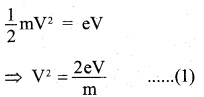When a magnetic field is applied normal to the path of electron, the force due to the filed makes the electron to move along the circular path. The radius of the circular path is given by,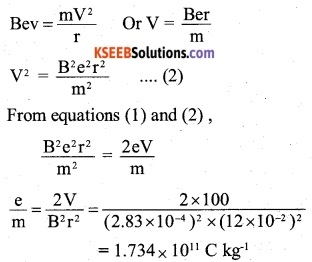Question 23.
(a) An X-ray tube produces a continuous spectrum of radiation with its short wavelength end at 0.45 Å. What is the maximum energy of a photon in the radiation?
(b) From your answer to (a), guess what order of accelerating voltage (for electrons) is required in such a tube?
λ= 0.45 A = 0.45 x 10-10 m
h = 6.62×10-34 Js
C = 3 x 108ms-1
(a) The maximum energy of the photon is given by,
$$E=\frac { hC }{ \lambda }$$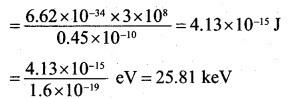(b) To produce electrons of energy 25.81 keV, accelerating potential of 25.81 kV i.e. order of 26 kV is required.Question 24.
In an accelerator experiment on high- energy collisions of electrons with positrons, a certain event is interpreted as annihilation of an electron-positron pair of total energy 10.2 BeV into two γ -rays of equal energy. What is the wavelength ‘ associated with each y-ray? (IBeV – 109 eV)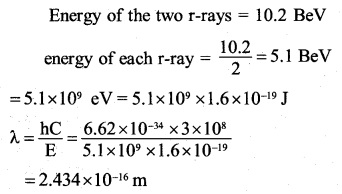Question 25.
Estimating the following two numbers should be interesting. The first number will tell you why radio engineers do not, need to worry much about photons! The second number tells you why our eye can never ‘count photons’, even in barely detectable light.

(a) The number of photons emitted per second by a Medium wave transmitter of 10 kW power, emitting radiowaves of wavelength 500 m.

(b) The number of photons entering the pupil of our eye per second corresponding to the minimum intensity of white light that we humans can perceive (~10-10 W m-2). Take the area of the pupil to be about 0.4 cm2, and the average frequency of white light to be about 6 x 1014Hz.
(a) Power of energy transmitted per second,
E = 10 kW = 104 W = 104 Js-1
λ = 500 m
Energy of one photon,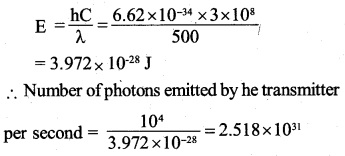(b) Minimum intensity of white light = 1010 Wm2
Area of the pupil = 0.4cm2 = 0.4 x 104 m2
∴ Light energy falling on pupil per second
= 10-10 x 0.4 x 10-4= 0.4 x 10-14W
Frequency of white light, u = 6x 1014 Hz
∴ Energy of the photon of the white light,
hV= 6.62×10-34 x 6 x 1014J
∴ Number of photons entering the pupil of our eye per second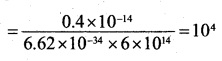Question 26.
Ultraviolet light of wavelength 2271 A from a 100 W mercury source irradiates a photo-cell made of molybdenum metal. If the stopping potential is -1.3 V, estimate the work function of the metal. How would the photo-cell respond to a high intensity (~ 10s W m-2) red light of wavelength 6328 A produced by a He-Ne laser?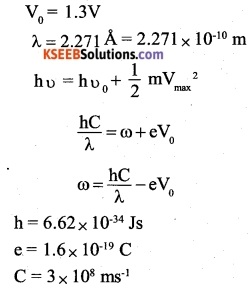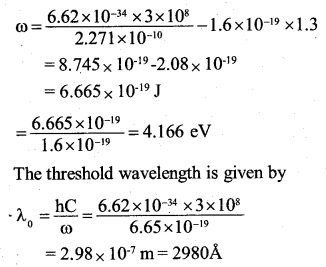Since wavelength 6.328 Å produced by He-Ne laser and incident on the photocell is greater than,λo the photo cell will not respond.

Question 27.
Monochromatic radiation of wavelength nm ( 1 nm = 10-9 m) from a neon lamp irradiates photosensitive material made of caesium on tungsten. The stopping voltage is measured to be 0.54 V. The source is replaced by an iron source and its 427.2 nm line irradiates the same photo-cell. Predict the new stopping voltage.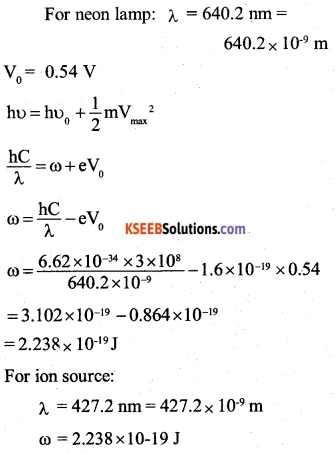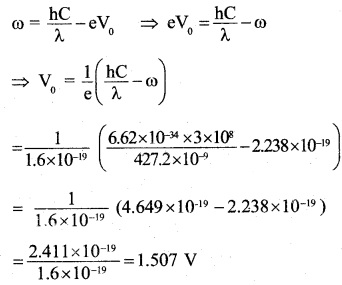Question 28.
A mercury lamp is a convenient source for studying frequency dependence of photoelectric emission, since it gives a number of spectral lines ranging from the UV to the red end of the visible spectrum. In our experiment with rubidium photo­cell, the following lines from a mercury source were used:
λ1= 3650 Å, λ2= 4047 A, λ3= 4358 A, λ4= 5461 A,λ5= 6907 A,
The stopping voltages, respectively, were measured to be:
V01 = 1.28 V, F02 = 0.95 V, Vn = 0.74 V, V04 = 0.16 V, Fos = 0 V
Determine the value of Planck’s constant h, the threshold frequency and work function for the material.
[Note: You will notice that to get h from the data, you will need to know e (which you can take to be 1.6 x 10-19 C). Experiments of this kind on Na, Li, K, etc. were performed by Millikan, who, using his own value of e (from the oil-drop experiment) confirmed Einstein’s photoelectric equation and at the same time gave an independent estimate of the value of h.
(a) Let the respective frequencies of the five spectral lines of mercury be υ1, υ2, υ3, υ4 and υ5. Then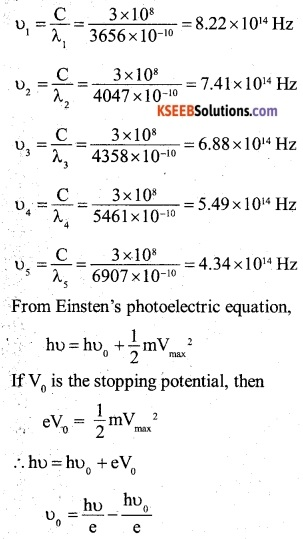It represents the equation of straight line, whose slope is hυ0/e and makes an intercept role on negative V0 – axis. The plot of graph between V (along x-axis) and V0 (along y-axis) for the given data for the five spectral lines will be shown as in figure.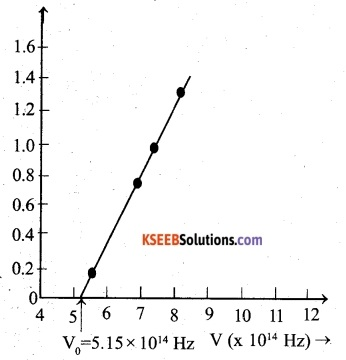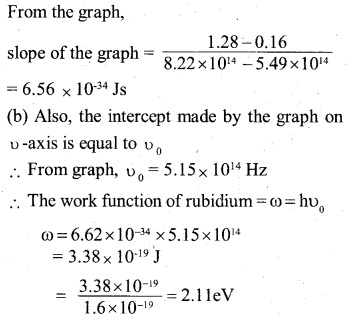Question 29.
The work function for the following metals is given: Na: 2.75 eV; K: 2.30 eV; Mo: 4.17 eV; Ni: 5.15 eV. Which of these metals will not give photoelectric emission for a radiation of wavelength 3300 Å from a He-Cd laser placed 1 m away from the photocell? What happens if the laser is brought nearer and placed 50 cm away?
λ. = 3300Å= 3300 ×10-10m
Energy of a photon of incident light,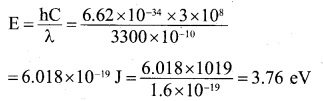Since the work functions of Mo and Ni are greater than the energy of the photon of incident light, photoelectric emission will not occur for these metals.

If the laser is brought nearer, the intensity of incident radiation on the metal will increase. It will increase the photoemission in case of Na and K. However, no photo electric emission will .take place in case of Mo and N.Question 30.
Light of intensity 10-5 W m-2 falls on a sodium photo-cell of surface area 2 cm2. Assuming that the top 5 layers of sodium absorb the incident energy, estimate time required for photoelectric emission in the wave-picture of radiation. The work function for the metal is given to be about 2 eV. What is the implication of your answer?
Since size of the atom is about 10-10m, the effective area of an atom may be considered as 10-20 m2.
Area of each layer in sodium = 2cm2 = 2 x 10-4m2
Number of atoms in 5 layers of sodium
$$\frac { 2\times { 10 }^{ -4 }\times 5 }{ { 10 }^{ -20 } }$$
If we assume that sodium has one conduction electron per atom, then number of electrons in the 5 layers of sodium = $${ 10 }^{ 17 }$$
Energy incident per second on the surface of the photocell
= 10-5 x 2 x 10-4
= 2×10-9
Since incident energy is observed by 5 layers of sodium, according to wave picture of radiation, the electrons present in all the 5 layers of sodium will share the incident equally.
∴ Energy received by any one electron in the 5 layers of sodium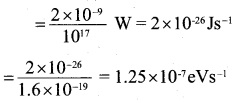Since work function of sodium is 2 eV, an electron will be ejected as soon as it gathers energy equal to 2 eV.
∴ Time required for photoelectric emission
= $$\frac { 2 }{ 1.25\times { 10 }^{ 7 } }$$ = 1.6 x 107s ≈ 0.5 year
It is contrary to the observed fact that there is no time lag between the incidence of light and the emission of photoelectrons.

Question 31.
Crystal diffraction experiments can be performed using X-rays, or electrons accelerated through appropriate voltage. Which probe has greater energy? (For quantitative comparison, take the wavelength of the probe equal to 1 A, which is of the order of inter-atomic spacing in the lattice) (me=9.11 ×10-31 kg).
For x- ray photon of wavelength of 1Å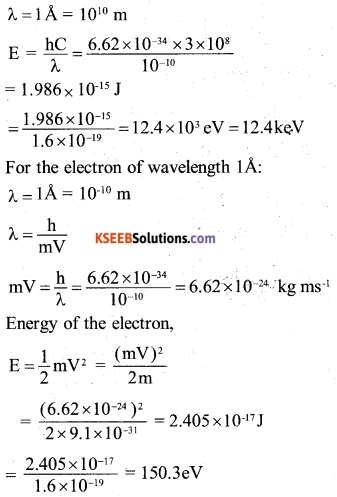Question 32.
(a) Obtain the de Broglie wavelength of a neutron of kinetic energy 150 eV. As you have seen in Exercise 11.31, an electron beam of this energy is suitable for crystal diffraction experiments. Would a neutron beam of the same energy be equally suitable? Explain. (mn = 1.675 x 10-27 kg)

(b) Obtain the de Broglie wavelength associated with thermal neutrons at room temperature (27 °C). Hence explain why a fast neutron beam needs to be thermalised with the environment before it can be used for neutron diffraction experiments.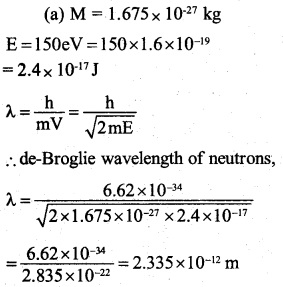Since the interatomic spacing (≈10-10m) is about
100 times greater than the de=Broglie wavelength of 150 eV neutrons, they are not suitable for crystal diffraction experiments.

(b)T=27+273=300k
Energy of the neutron at temperature, T,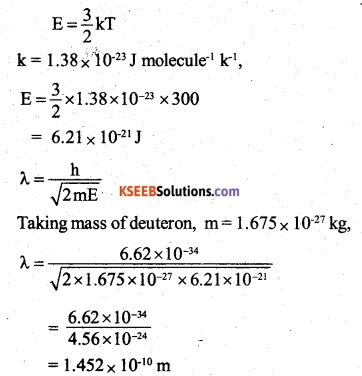Thus, thermal neutrons have wavelength of the order of interatomic spacing
(≈10-10m) and therefore they are suitable for diffraction experiments. Fast neutrons will possess wavelength quite small as compared to interatomic spacing. Hence for neutron diffraction experiments, fast neutron beam needs to be thermalised.

Question 33.
An electron microscope uses electrons accelerated by a voltage of 50 kV. Determine the de Broglie wavelength associated with the electrons. If other factors (such as numerical aperture, etc.) are taken to be roughly the same, how does the resolving power of an electron microscope compare with that of an optical microscope which uses yellow light?
Here, V = 50 kV
Therefore, energy of electrons,
E = 50keV = 50 x 103 x 1.6 x 1019 =80 x 10-15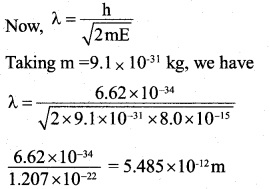The resolving power of a microscope is inversely proportional to the wave length of the radiation used. Since wavelength of the yellow light is 5,990 A i.e. 5.99 x 10-7 m, it follows that the electron microscope will possess resolving power 10 times as large as that of the optical microscope.Question 34.
The wavelength of a probe is roughly a measure of the size of a structure that it can probe in some detail. The quark structure of protons and neutrons appears at the minute length-scale of  10-15m or less. This structure was first probed in early 1970’s using high energy electron beams produced by a linear accelerator at Stanford, USA. Guess what might have been the order of energy of these electron beams. (Rest mass energy of electron = 0.511 MeV.)
As the quark structure of protons and neutrons appears a the length-scale of 10-15m, the electron beam used should be of the wavelength of this order i.e.
λ= 10-15m
Therefore, momentum of the electron beam,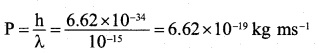The electron having momentum of this order possesses speed comparable to the speed of light. Therefore, its energy can be found by using the relativistic formula for die total energy of the electron i.e.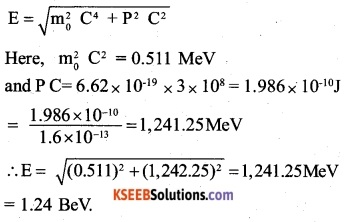Question 35.
Find the typical de Broglie wavelength associated with a He atom in helium gas at room temperature (27 °C) and 1 atm pressure; and compare it with the mean separation between two atoms under these conditions.
Mass of the He-atom,
$$m=\frac { atomic\quad weight\quad of\quad He }{ Avogadro\quad Number } \frac { 4 }{ 6.02\times { 10 }^{ 23 } }$$
= 6.645 × 10-24 g = .6645 ×10-27 kg
By proceeding as in solved problem No.2.09,
find the de-Broglie wavelength of the He-atom at 27°C.
It can be obtained that de-Broglie wavelength of the He-atom,
λ= 0.73 x 10-10 m = 0.73 Å
If V is volume of the gas and dj the mean separation between two atoms in the gas, then V = d3 N,
From the perfect gas equation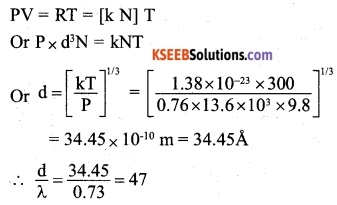i.e the separation between the two He-atoms in the gas is much larger than the Broglie wavelength of the atom.

Question 36.
Compute the typical de Broglie wavelength of an electron in a metal at 27 °C and compare it with the mean separation between two electrons in a metal which is given to be about 2 x 10-10 [Note: Exercises 11.35 and 11.36 reveal that while the wave-packets associated with gaseous molecules under ordinary conditions are non-overlapping, the electron wave-packets in a metal strongly overlap with one another. This suggests that whereas molecules in an ordinary gas can be distinguished apart, electrons in a metal cannot be distinguished apart from one another. This indistinguishability has many fundamental implications which you will explore in more advanced Physics courses.]
It cap be obtained that de-Broglie wavelength of the electron,
λ – 62.3 x 10-10 m = 62.3 A
Mean separation between two electrons in the metal,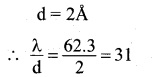i.e de-Broglie wavelength of the electron is much larger than the separtation between two electrons in the metal.Question 37.

(a) Quarks inside protons and neutrons are thought to carry fractional charges [(+2/3)e ; (-1/3)e]. Why do they not show up in Millikan’s oil-drop experiment?
(b) What is so special about the combination e/m? Why do we not simply talk of e and m separately?
(c) Why should gases be insulators at ordinary pressures and start conducting at very low pressures?
(d) Every metal has a definite work function. Why do all photoelectrons not come out with the same energy if incident radiation is monochromatic? Why is there an energy distribution of photoelectrons?
(e) The energy and momentum of an electron are related to the frequency and wavelength of the associated matter wave by the relations:
$$\frac { h }{ \lambda }$$
But while the value of x is physically significant, the value of v (and therefore, the value of the phase speed v X) has no physical significance. Why?
(a) Quarks having the fractional charges are thought to be confined within a proton or a neutron only. These quarks are bound by forces, which grow stronger, if they are tried to be pulled apart. Thus, the quarks always remain together. It follows that though fractional v charges exist in nature, the observable charges are always integral multiples of e.

(b) The motion of an electron inside electric and magnetic fields is governed by
eV = $$\frac { 1 }{ 2 }$$-mv2 = eV; eE = ma and B eV =$$\frac { m{ v }^{ 2 } }{ r }$$ where the symbols have their usual meanings.
In all the three relations, e and m occur separately. Further, the value of a physical variable involved in any of these reations can be found by substituting the value of e/m.

(c) The gases keep on getting ionised due to energetic rays [X-rays, Cosmic rays, etc.,] passing through them.

However, at atmospheric pressure, the positive and negative ions are too close and recombine to form neutral gas atoms. Due to this, the gases behave as insulatiors at ordinary pressure.

At low pressures, the gas ions do not recombine as they are appreciably apart. Due to the presence of free ions, the gases start conducting at low pressures.

(d) Work function of a metal is the minimum energy required to knock out an electron (from the conduction band). Since electrons are present in a continuous band of levels, different electrons require different amounts of energy to get out of the atom. For this reason electrons knocked off by a monochromatic radiation posses different energies.

(e) Whereas energy E (= hv) of a particle is arbitrary to within an additive constant, its momentum p (= h/ λ) is not. So, for the matter wave associated with an electron the wavelength λ is physically significant and its frequency v, is not so likewise, the phase speed v λ, is also not physically significant.

Question 1.
An electron and an alpha particle have the same de-broglie wavelength associated with them. How are their kinetic energies related to each other?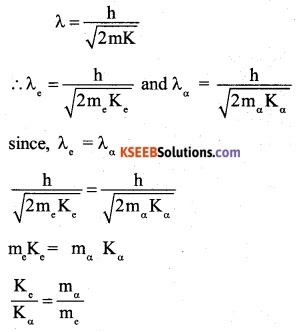Question 2.
If the frequency of the incident radiation is equal to the threshold frequency, what will be the value of the stopping potential?
From Einstein’s photoelectric equation,
hv = hv0 + eV0
v = v0
hv0=hv0 + eV0
v0=o
∴ Stopping potential = 0

Question 3.
It is harder to remove a free electron from copper than from sodium. Which metal has greater work function? Which has higher threshed wavelength?
As it is harder to remove an electron from copper than sodium, the work function of copper is greaterAs threshold wavelength is inversely proportional to the work function, its value will be more for sodium.

Question 4.
An electromagnetic wave of wavelength X is incident on a photosensitive surface of negligible work function. If the phot- electrons emitted from this surface have de-Broglie wavelength λ1 prove that $$\lambda =\frac { 2mc }{ h } { \lambda }_{ 1 }^{ 2 }$$
Since photosensitive surface of negligible work function, K.E of emitted electron = energy of incident photon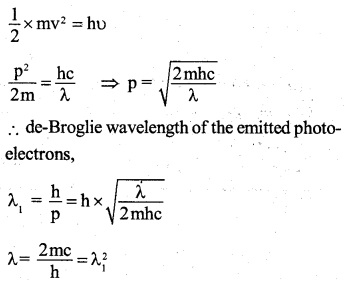Question 5.
Find the momentum of an x-ray photon of wavelength.
Let p be the momentum of the x-ray photon of wavelength x, then energy of x-ray photon,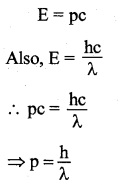Question 6.
The work function of a substance is 4eV. The longest wavelength of light that can cause photoelectric emission from this substance is approximately
(A) 540 nm
(B) 400 nm
(C) 310 nm
(D) 220 nm
(C) 310 nm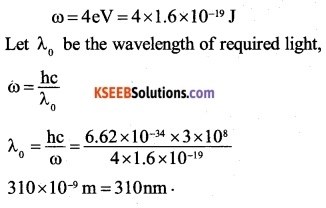Question 7.
The mass of a photon at rest is
(A) zero
(B) 1.67 x 10-35kg
(C) 1 amu
(D) 9 x 1031 kg
(A) zero

Question 8.
Which one among the following shows particle nature of light?
(A) Photoelectric effect
(B) Interference
(C) Refraction
(D) Polarisation
(A) Photoelectric effectQuestion 9.
Planck’s constant has the dimensions of
(A) linear momentum
(B) angular momentum
(C) energy
(D) power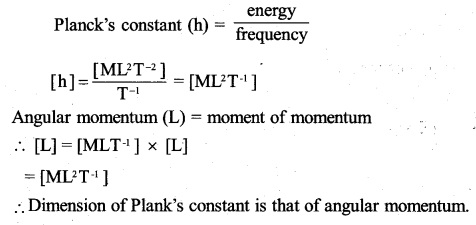(A) $$\frac { h }{ mv }$$
(C) $$\frac { mh }{ v }$$
(D) $$\frac { m }{ hv }$$